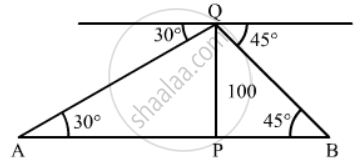Share

# From the Top of a Tower, 100, High, a Man Observes Two Cars on the Opposite Sides of the Tower and in Same Straight Line with Its Base, with Angles of Depression 30° and 45°. Find the Distance Between the Cars - CBSE Class 10 - Mathematics

#### Question

From the top of a tower, 100, high, a man observes two cars on the opposite sides of the tower and in same straight line with its base, with angles of depression 30° and 45°. Find the distance between the cars. [Take sqrt3 = 1.732]

#### SolutionLet PQ be the tower and A and B are two cars.
We have,

PQ = 100 m, ∠PAQ = 30° and ∠PBQ = 45°

In ∆APQ

tan 30^@ = (PQ)/(AP)

=> 1/sqrt3 = 100/(AP)

=> AP =100sqrt3 m

Also In ∆ BPQ

tan 45^@ = (PQ)/(BP)

=> 1= 100/(BP)

=> BP = 100 m

Now, AB = AP + BP

= 100sqrt3 + 100

=100(sqrt3 + 1)`

=100 x (1.732 + 1)

= 100 x 2.732

= 273.2 m

So the distance between the cars is 273.2 m

Is there an error in this question or solution?

#### Video TutorialsVIEW ALL 

Solution From the Top of a Tower, 100, High, a Man Observes Two Cars on the Opposite Sides of the Tower and in Same Straight Line with Its Base, with Angles of Depression 30° and 45°. Find the Distance Between the Cars Concept: Heights and Distances.
S